# Numeric Classification

 Tip Because numbers can be represented as binary values using only two symbols (0 and 1), it is possible to use an ANN to classify numbers. In this case, each ANN input will be used for one bit in the number. The binary number can be represented in BCD, gray code or any code.

 Problem 1 Create a New Project called Div57 (You must select: Multi-layer Network and Classification in the New Project Dialog) as shown below to classify the numbers that are divisible by 5 or by 7. Edit the BuilTrainSet.lab file to build an appropriate training set for the classification of the numbers that are divisible by 5 or by 7. The classifier should be able to classify any number from 0 to 31 (5 bits). The input training set must include 16 times each number. The classifier must be able to tolerate inputs contaminated with 10% of noise. Figure (a) shows the classifier behavior when number 5 (base 10) is applied to the network; output 1 is one while output 2 is zero. Figure (b) shows the neural network when number 7 (base 10) is at the input of the classifier; output 1 is zero and output 2 is one. In figure (c) the input is number 1; both outputs are zero.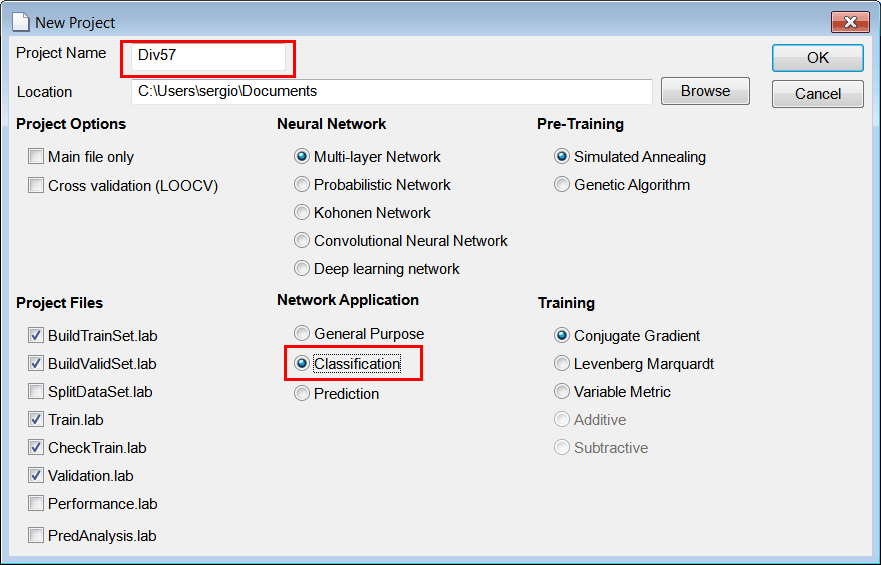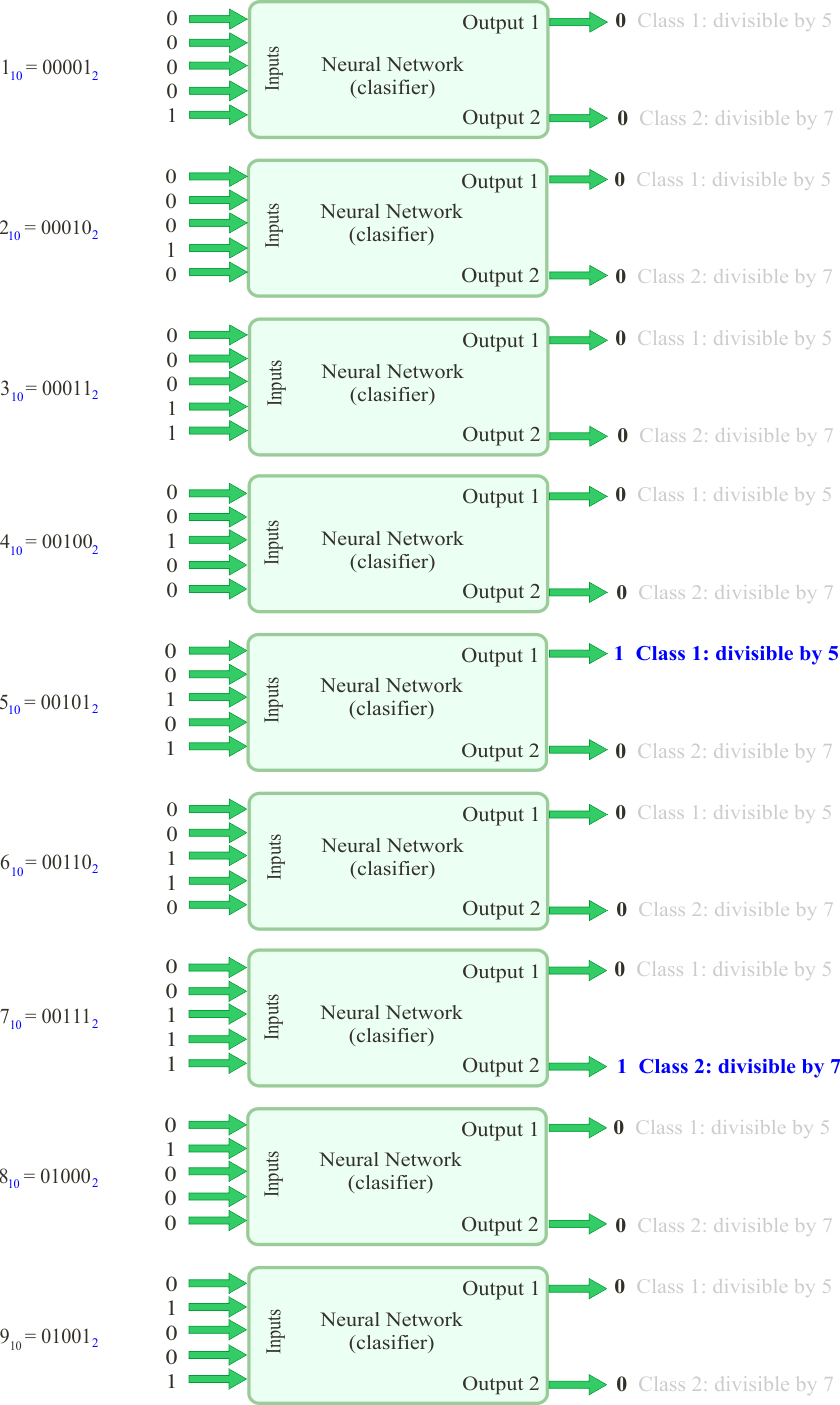Solution 1 After editing the file,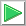Runclick the button to execute the code. If you do not have any errors, the training set will be generated and displayed on the variable list and the file list. Click on the file to see its contents. The first 32 rows of the training set are the binary representation of the numbers from 0 to 31; the second 31 rows of the training set are again the representation of the number from 0 to 31. The training set target has two columns, the first column is for class 1 (divisible by 5) and the second column is for class 2 (divisible by 7).

 Div57\BuildTrainSet.lab Matrix trainSetTarget; trainSetTarget.Create(32, 2); // Class1: divisible by 5, Class 2: divisible by 7 Matrix input; Matrix column; Matrix row; Vector number; int i = 0; for(i = 0; i<32; i++) {      number.CreateBinary(5, i); // Convert to binary      column = number; // Binary number in one column      row = column.Transpose(); // Binary number in one row      input.AppendDown(row);      if (i%5 == 0 && i != 0) // Is divisible by 5 (class 1)?      {           trainSetTarget[i] = 1;      }      if (i%7 == 0 && i != 0) // Is divisible by 7 (class 2)?      {           trainSetTarget[i] = 1;      } } input.AppendDown(input); input.AppendDown(input); input.AppendDown(input); input.AppendDown(input); input.Save(); // Save the input to create the validation set // Matrix noise; noise.CreateRandom(input.GetRowCount(), input.GetColCount(), 0.0, 1.0); Matrix trainSetInput = 0.9*input + 0.1*noise; trainSetInput.Save(); // trainSetTarget.AppendDown(trainSetTarget); trainSetTarget.AppendDown(trainSetTarget); trainSetTarget.AppendDown(trainSetTarget); trainSetTarget.AppendDown(trainSetTarget); trainSetTarget.Save();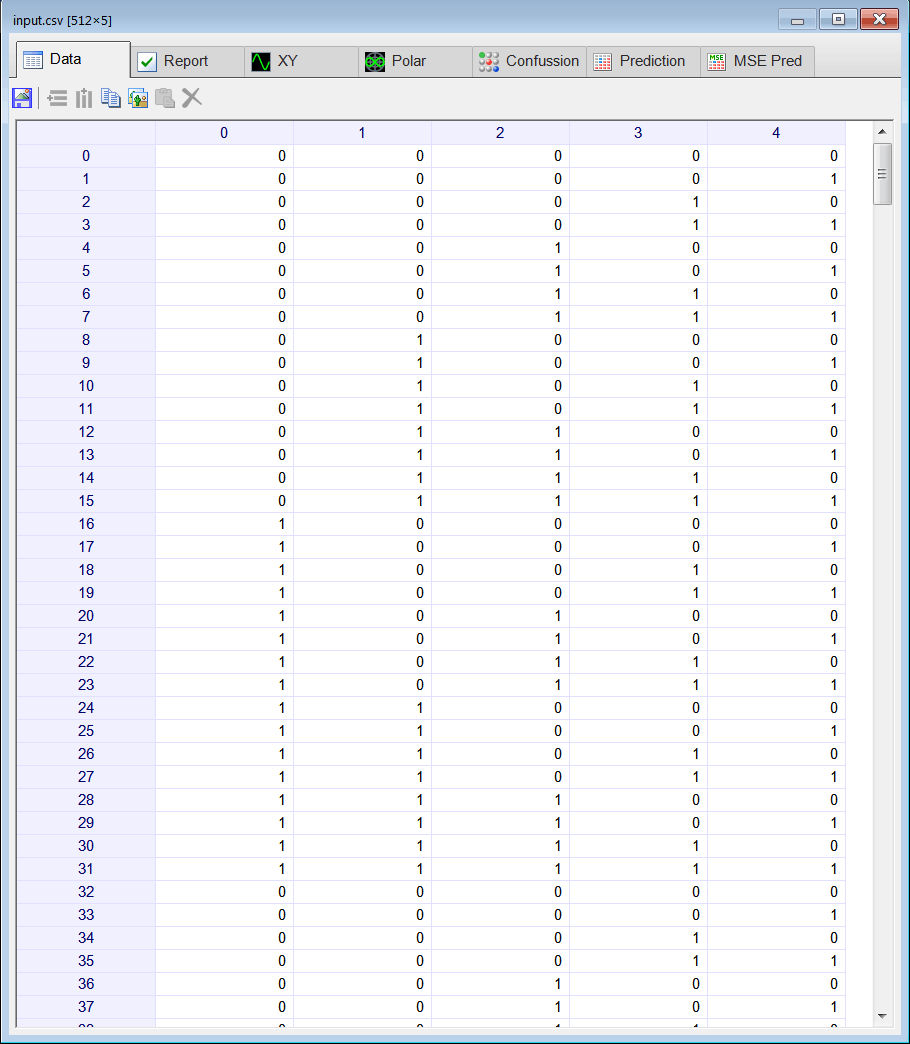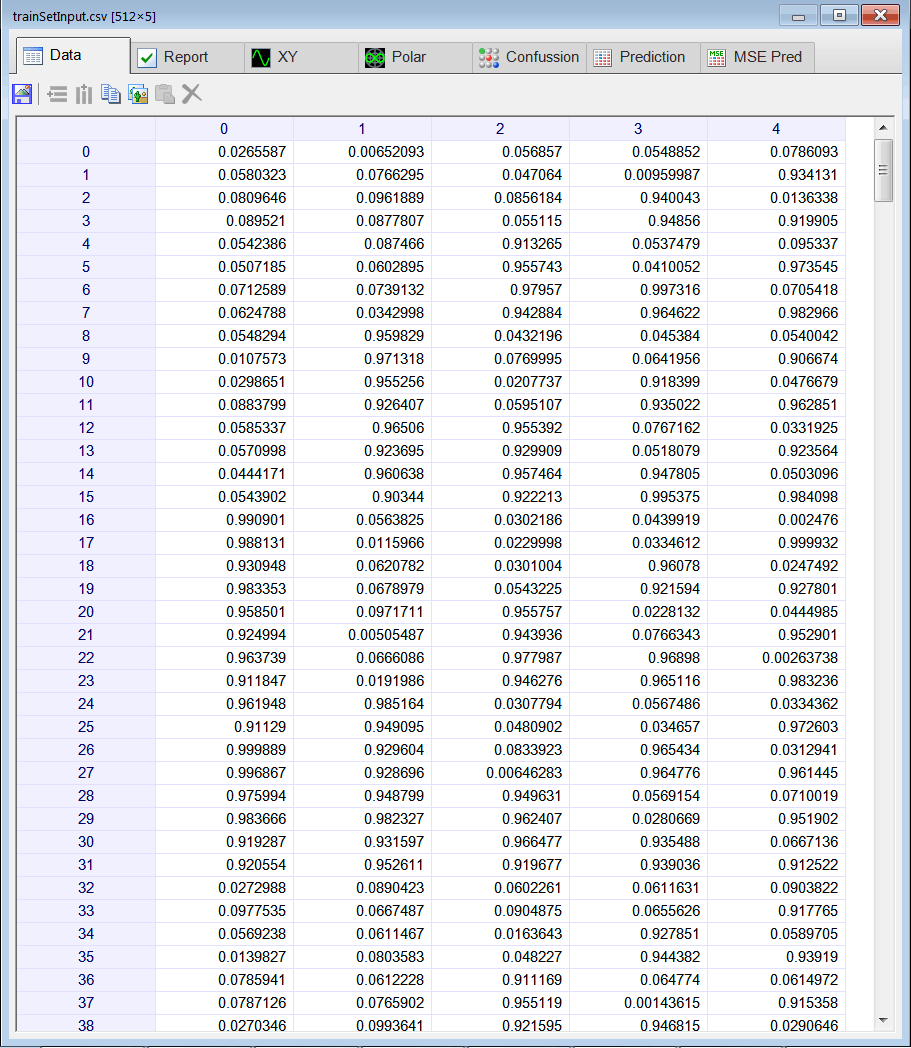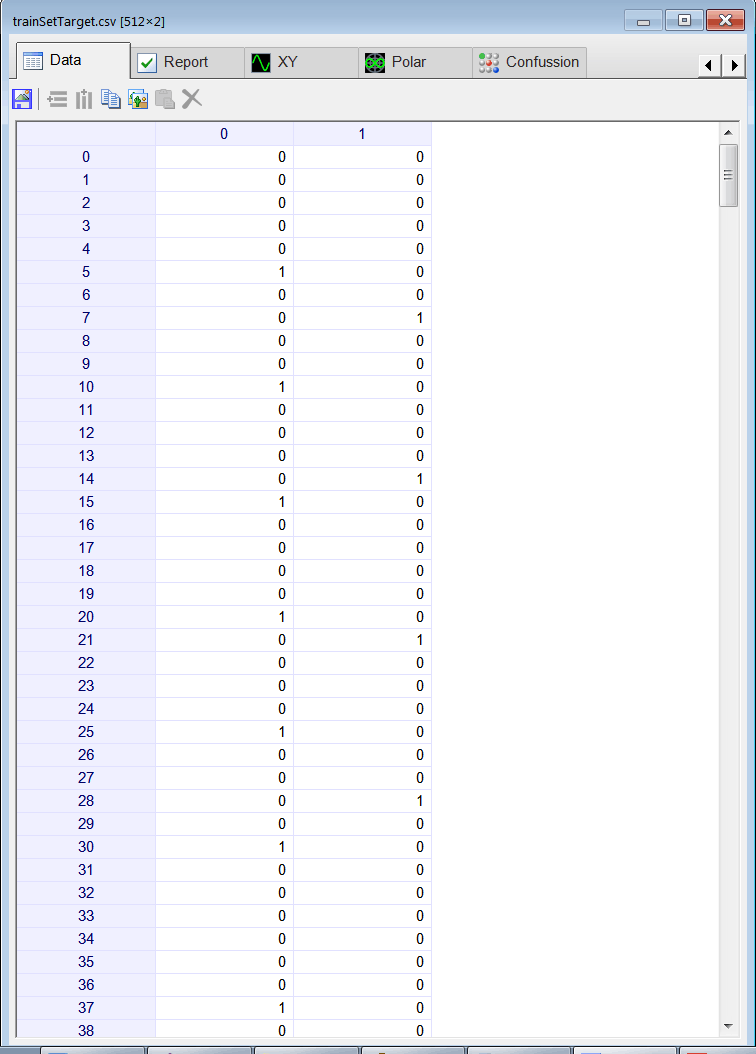Problem 2 Edit the BuilValidSet.lab file to build an appropriate validation set for problem 1.

 Solution 2 After editing the file,Run click the button to execute the code. If you do not have any errors, the validation set will be generated and displayed on the variable list and the file list. Use the scroll bar at the top to visualize each validation case. In this case the validation case was created in the same way than the training set was created; however, the additive noise creates a different data set.

 Div57\BuildValidSet.lab Matrix input; input.Load(); // Matrix noise; noise.CreateRandom(input.GetRowCount(), input.GetColCount(), 0.0, 1.0); Matrix validSetInput = 0.9*input + 0.1*noise; validSetInput.Save();

 Problem 3 Edit the Train.lab file to design and train an ANN for the classification of numbers that are divisible by 5 or divisible by 7. Use one hidden layer and adjust the number of neurons in this layer. Train the ANN using simulated annealing and the Levenberg Marquardt method.

 Solution 3 After editing the file,Run click the button to execute the code. If you do not have any errors, the ANN will be trained. Wait until the code execution stops. Double click the network in the variable list to see the network. Then, select the inputTrainSet.csv file and, the trainSetTarget.csv file. You will be able to see the network behavior in real time by moving the scrollbar at the right.

 Div57\Train.lab //_________________________ Network Setup LayerNet net; net.Create(5, 11, 0, 2); // 2 Outputs means 2 Classes //____________________________ Input Scaling net.SetInScaler(0, 0.0, 1.0); net.SetInScaler(1, 0.0, 1.0); net.SetInScaler(2, 0.0, 1.0); net.SetInScaler(3, 0.0, 1.0); net.SetInScaler(4, 0.0, 1.0); //____________________________ Output Scaling net.SetOutScaler(0, 0.0, 1.0); net.SetOutScaler(1, 0.0, 1.0); //________________________ Load and set the training set Matrix trainSetInput; trainSetInput.Load(); Matrix trainSetTarget; trainSetTarget.Load(); net.SetTrainSet(trainSetInput, trainSetTarget, false); //________________________ Train net.TrainSimAnneal(100, 100, 15, 0.001, false, 4, 1.0e-12); net.TrainLevenMar(2000,1.0e-12); //_____________________________ Save the trained network net.Save();

 Tip In the figure below the input of the network is number 5, observe that the value in output 1 is one while the value in output 2 in zero as expected.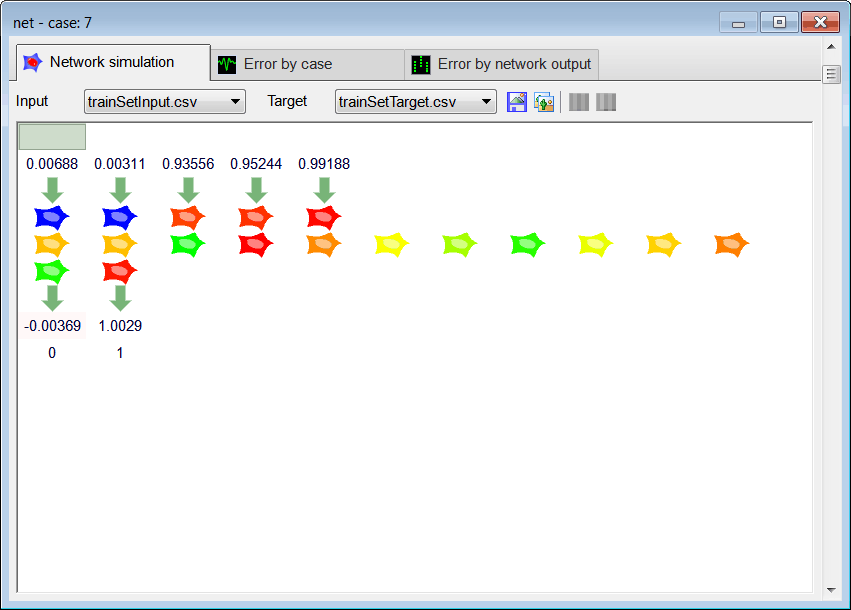Problem 4 Edit the CheckTraining.lab file to check the training: (a) Compute the confusion matrix using the training set. (b) Plot the error for each network output. (c) Save the confusion matrix as a vector image (checkTraining.emf).

 Solution 4 (a) After editing the file,Run click the button to execute the code. If you do not have any errors, the number of errors will be displayed in the variable list. Click the variable called trainConf to view the confusion matrix for the training set, it should have zero errors as shown below. The numbers that are divisible by 5 are 5, 10, 15, 20, 25 and 30, that is 6x16, 96 elements in class 1. The numbers that are divisible by 7 are 7, 14, 21 and 28, that is 4x16, 64 elements in class 2. From the total number of training cases (512), we can deduct the 96 elements in class 1 and the 64 elements in class 2; this will result in 352 cases that do not belong to class 1 neither to class 2.

 Div57\CheckTraining.lab //_________________________________________ Load the training set Matrix trainSetInput; trainSetInput.Load(); Matrix trainSetTarget; trainSetTarget.Load(); //_________________________________________ Load the ANN LayerNet net; net.Load(); //_________________________________________ Run Matrix output = net.Run(trainSetInput); //_________________________________________ Compute the confusion matrix Matrix trainConf = ConfusionMatrix(output, trainSetTarget, 0.5); trainConf.Save(); //_________________________________________ Compute the Number of Errors int numErrors = toint(trainConf.GetSum()) - toint(trainConf.GetDiagonalSum());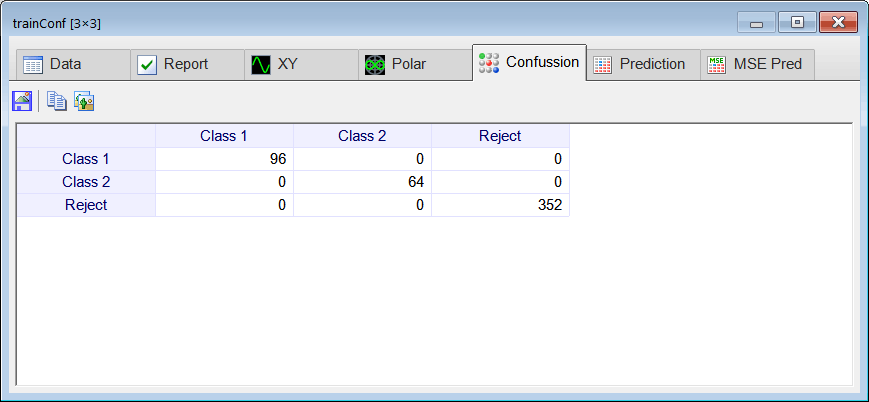Tip The last argument of the ConfusionMatrix is the threshold. Ideally it should be 0.5, however, you may try other values to reduce the number of errors. You must use the same threshold for training and for validation.

 Problem 5 Edit the Validation.lab file to perform the validation of the ANN. (a) Compute the confusion matrix using the validation set. (b) Plot the error for each network output. (c) Save the confusion matrix as a vector image (Validation.emf).

 Solution 5 (a) After editing the file,Run click the button to execute the code. If you do not have any errors, the number of errors will be displayed in the variable list. Click the variable called validConf to view the confusion matrix for the validation set, it should have zero errors (all elements outside the main diagonal must be zero).

 Div57\Validation.lab //_________________________________________ Load the validation set Matrix validSetInput; validSetInput.Load(); Matrix trainSetTarget; trainSetTarget.Load(); //_________________________________________ Load the ANN LayerNet net; net.Load(); //_________________________________________ Run Matrix output = net.Run(validSetInput); //_________________________________________ Compute the confusion matrix Matrix validConf = ConfusionMatrix(output, trainSetTarget, 0.5); validConf.Save(); //_________________________________________ Compute the Number of Errors int numErrors = toint(validConf.GetSum()) - toint(validConf.GetDiagonalSum());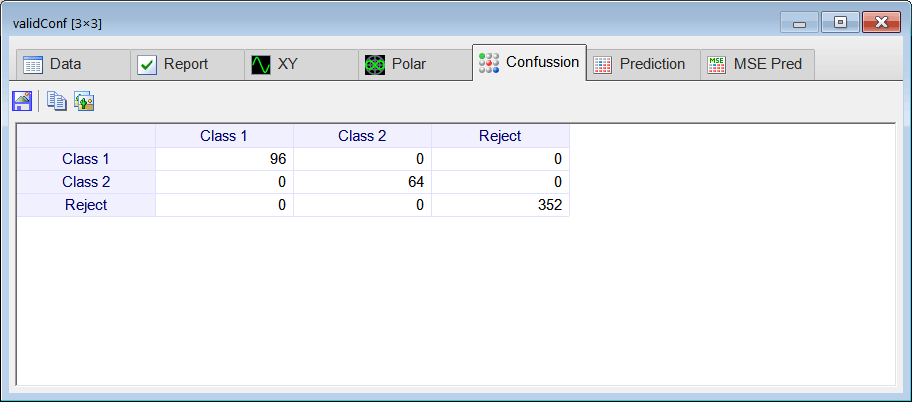Problem 6 Generate a report in Microsoft Word. Write some conclusions in the report focusing on the problems that were faced during the simulation and how these problems were or could be solved.